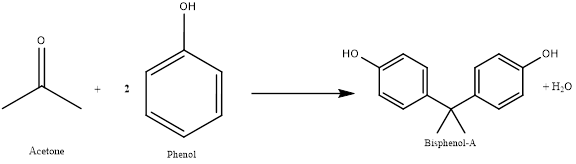Chapter 23.5, Problem 3.1ACP

Chapter
Section
Textbook Problem

What is the atom economy for the reaction of acetone with phenol to make BPA? (Applying Chemical Principles, 4.1: Green Chemistry and Atom Economy, page 207.)

Interpretation Introduction

Interpretation: The atom economy is to be calculated for the reaction of acetone and phenol to give BPA.

Concept introduction:

The synthesis of BPA is done by using acetone and phenol in the presence of an acid. This is type of friedal craft acylation reaction. The acetone molecule in presence of acid act as an electrophile. The reaction is written as,For any reaction atom economy is calculated by using the following expression,

Atom Economy = (Mass of desired productMass of all products)×100 (1)

Explanation

The value of atom economy for the reaction is calculated below.

Given:

In the given reaction two moles of phenol are reacting with one mole of acetone and giving one mole of BPA and a side product water.

The molar mass of BPA is 228 gmol1.

The molar mass of water is 18 gmol1.

Therefore, total mass of the product will be equal to the sum of molar mass of BPA and water.

Mass of all products is 246 gmol1.

As desired product is BPA, then substitute the values in equation (1)

Still sussing out bartleby?

Check out a sample textbook solution.

See a sample solution

The Solution to Your Study Problems

Bartleby provides explanations to thousands of textbook problems written by our experts, many with advanced degrees!

Get Started

Two tablespoons of peanut butter offer about the same amount of protein as a hot dog. T F

Nutrition: Concepts and Controversies - Standalone book (MindTap Course List)

How did the Ptolemaic model explain retrograde motion?

Horizons: Exploring the Universe (MindTap Course List)

In what way are the substrate and active site of an enzyme related?

Chemistry for Today: General, Organic, and Biochemistry